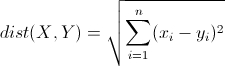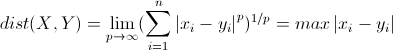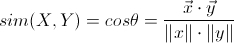KNN算法及利用KNN检测异常操作

1 KNN算法

1.1 KNN简介

K近邻算法的核心思想可以用一句很多文化中都有的古语概括：近朱者赤近墨者黑。评价一个人，一般从它的朋友就可以得知，因为一般和小混混整天呆在一起的就是小混混，和小学生一起玩的一般也是个小学生。KNN的基本思路也是这样，如果一个待分类的样本在样本空间中的K个最相近的样本中的大多数属于某个类别，那么该样本也属于这个类别。

KNN直接作用于带标记的样本，属于有监督的算法。

1.2 距离度量

KNN算法做分类任务时，需要对样本的相似度进行判断。“距离”和“相似系数”就是对相似度的两种度量方式，它是机器学习的核心概念之一，这里介绍一下几个常用的距离计算方式。

1.2.1 欧式距离>>> import numpy as np
>>> from math import *
>>> x = np.array([1,2])
>>> y = np.array([4,6])
>>> sqrt(sum(pow(x-y,2) for x,y in zip(x,y)))
5.0

1.2.2 曼哈顿距离>>> sum(abs(x-y))
7

1.2.3 切比雪夫距离>>> abs(x-y).max()
4

1.2.4 夹角余弦距离>>> np.dot(x,y)/(np.linalg.norm(x)*np.linalg.norm(y))
0.9922778767136677

1.3算法过程

• 1 计算距离：给出待分类的样本，计算样本和其他样本的距离
• 2 距离排序：将计算出的距离排序
• 3 选择k个相邻：找出k个距离最近的样本
• 3 决定分类：找出k个距离最近样本的主要分类，作为此待分类样本的类别

1.4 要素

KNN算法的分类结果由3个基本要素组成：距离度量、K的选择和分类决策。

2 利用KNN算法检测异常操作

2.1 数据搜集

Schonlau在他的个人网站：http://www.schonlau.net/上发布了针对Linux操作的训练数据。我们直接下载即可。2.2 数据特征化

• 第一步：对日志中所有操作命令使用次数进行排序，选出使用最多的前50条记做M1，选出使用最少的前50条记做M2。
• 第二步：每100条操作命令组成一个操作序列，这样的序列有150个，这100条操作中不重复的命令个数，记做F1。100条操作中最多的10条且在M1中的命令个数（重合度），记做F2 。这100条操作中最少的10条且在M2知乎的命令个数（重合度），记做F3 。一个序列{F1,F2,F3}就作为这个序列的特征，这样的特征有150个，前面120个作为训练集，后面30个作为测试集。
• 第三步：打标签，前50个正常全部是0，后100个有0也有1。后100条从lable.txt中读取，user3就对应第三列

import operator
from nltk import FreqDist

cmd_list=[]
dist=[]
with open(filename) as f:
i=0
x=[]
for line in f:
line=line.strip('\n')
x.append(line)
dist.append(line)
i+=1
if i == 100:
cmd_list.append(x)
x=[]
i=0

fdist = sorted(FreqDist(dist).items(),key = operator.itemgetter(1),reverse = True)
dist_max = [item for item in fdist[:50]]
dist_min = fdist[-50:]
return cmd_list,dist_max,dist_min
# cmd_list包含150组数据，每组100个命令，所以一共是15000个命令。
# dist_max 最常用的50个命令
# dist_min 最不常用的50个命令

def get_user_cmd_feature(user_cmd_list,dist_max,dist_min):
user_cmd_feature = []
for cmd_block in user_cmd_list:
f1 = len(set(cmd_block))
fdist = sorted(FreqDist(cmd_block).items(),key = operator.itemgetter(1),reverse = True)
f2=fdist[0:10]
f3=fdist[-10:]
f2 = len(set(f2) & set(dist_max))
f3=len(set(f3) & set(dist_min))
x=[f1,f2,f3]
user_cmd_feature.append(x)
return user_cmd_feature

def get_label(filename, index = 0):
x=[]
with open(filename) as f:
for line in f:
line=line.strip('\n')
x.append(int(line.split()[index]))
return x

if __name__ =="__main__":
user_cmd_feature = get_user_cmd_feature(user_cmd_list,user_cmd_dist_max,user_cmd_dist_min)
y = *50+labels
N = 120
x_train=user_cmd_feature[0:N]
y_train=y[0:N]
x_test=user_cmd_feature[N:150]
x_test=y[N:150]

2.3 KNN训练

from sklearn.neighbors import KNeighborsClassifier
from sklearn.metrics import accuracy_score

if __name__ =="__main__":
knn = KNeighborsClassifier(n_neighbors=3) # 创建分类器，这里algorithm采用'auto'，会在学习时自动选择最合适的算法
knn.fit(x_train,y_train) # 训练
predictions = knn.predict(x_test) # 测试
print(accuracy_score(y_test, predictions)) # 验证

2.3 改进

cmd_list=[]
dist=[]
with open(filename) as f:
i=0
x=[]
for line in f:
line=line.strip('\n')
x.append(line)
dist.append(line)
i+=1
if i == 100:
cmd_list.append(x)
x=[]
i=0
fdist = list(FreqDist(dist).keys())
# fdist 去重后的全部命令列表
# cmd_list包含150组数据，每组100个命令，所以一共是15000个命令。
return cmd_list,fdist

def get_user_cmd_feature_new(user_cmd_list,dist):
user_cmd_feature=[]
for cmd_list in user_cmd_list:
v=*len(dist)
for i in range(0,len(dist)):
if dist[i] in cmd_list:
v[i]+=1
user_cmd_feature.append(v)
return user_cmd_feature
user_cmd_feature = get_user_cmd_feature_new(user_cmd_list,dist)

y = *50+labels
N = 100

x_train=user_cmd_feature[0:N]
y_train=y[0:N]
x_test=user_cmd_feature[N:150]
y_test=y[N:150]
knn = KNeighborsClassifier(n_neighbors = 3) # 创建分类器
knn.fit(x_train,y_train) # 训练

predictions = knn.predict(x_test) # 测试
print(predictions)

3 参考

• 《python机器学习实战》
• 《web安全机器学习入门》
1 + 2 =